MTH4140 Home

# MTH4140: Midterm 1 Practice 3

Problem 1

Which of the following statements are true?

• (A) If $$R$$ is a relation on $$A$$, then $$R\times R = A\times A\times A\times A$$.

• (B) A transitive relation on the set $$S$$ must be a subset of $$S\times S$$.

• (C) If $$f:A\to A$$ is a function then the set $$V=\left\{\left(x,f(x)\right):x\in A\right\}$$ is a relation on $$A$$.

• (D) If $$P$$ is a reflexive relation on $$Q$$ then $$Q$$ is a symmetric relation on $$P$$.

• (E) If $$P$$ is a relation on $$Q$$ then $$P\times Q$$ is also a relation on $$Q$$.

Problem 2

Provide an example of a set $$S$$ of $$3$$ elements and a function $$f:S\times S\to S$$ that is not surjective.

Problem 3

Determine which pairs of graphs below are isomorphic.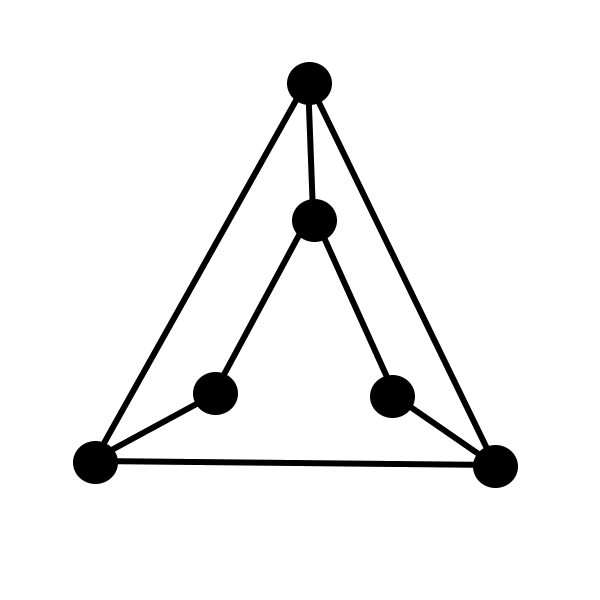$$\quad$$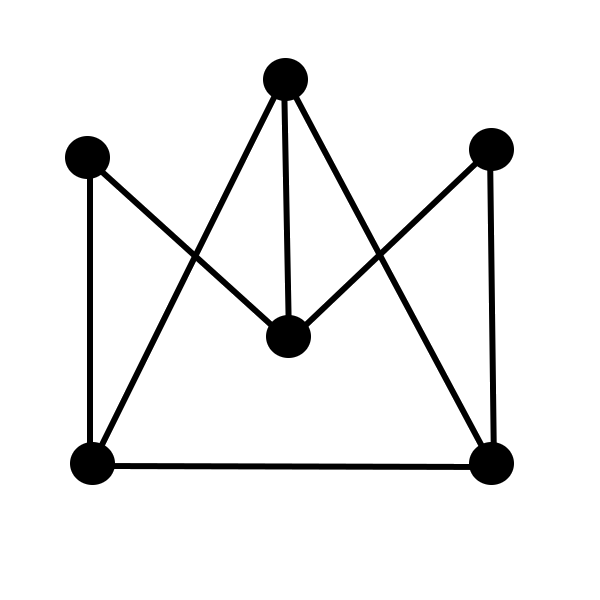$$\quad$$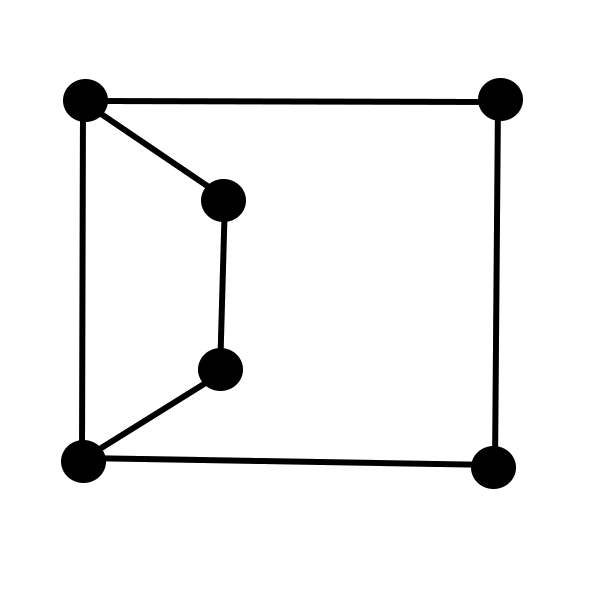Problem 4

Which of the pictures below can be drawn without lifting the pen from the paper and without tracing the same line twice?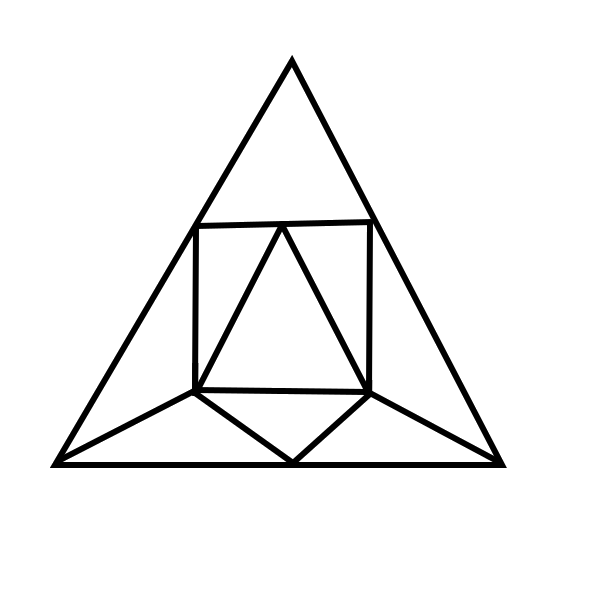$$\quad$$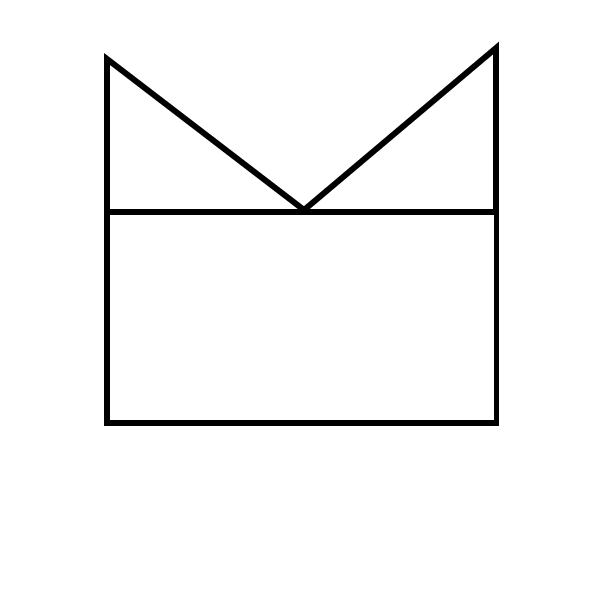$$\quad$$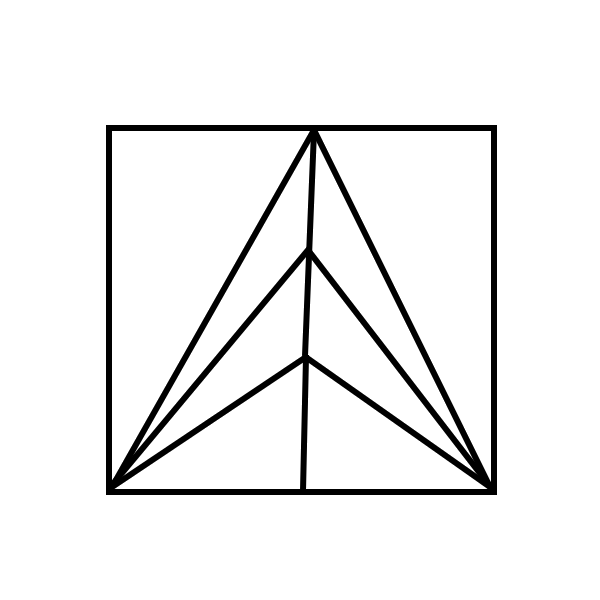Problem 5

A sequence of integers $$\left(a_n\right)_{n=1}^{\infty}$$ satisfies $$a_1=6$$, $$a_2=20$$, and $$a_{n+2}=2a_{n+1}+8a_n$$ for $$n\geq 1$$. Prove that $a_n=\frac{4 \cdot 4^n-{ } \left(-2\right)^n }{3} \quad\mbox{ for every } n\geq 1.$

Problem 6

Assume that the set $$V$$ is defined as $$V =\left\{ 1,2,\dots, 43 \right\}^{6}$$. Assume that $$E$$ is the set of all pairs $$(u,v)$$ such that $$u=\left(a_1, \dots, a_{6}\right)$$ and $$v=\left(b_1,b_2, \dots, b_{6}\right)$$ are elements of $$V$$ for which the number $$\left(a_1-b_1\right)\cdot\left(a_2-b_2\right) \cdots \left(a_{6}-b_{6}\right)$$ is not divisible by $$7$$. Determine whether the graph $$(V,E)$$ has a path that visits every edge exactly once.